Courses

# Test: Chirality Isomerism

## 24 Questions MCQ Test Chemistry for JEE | Test: Chirality Isomerism

Description
This mock test of Test: Chirality Isomerism for JEE helps you for every JEE entrance exam. This contains 24 Multiple Choice Questions for JEE Test: Chirality Isomerism (mcq) to study with solutions a complete question bank. The solved questions answers in this Test: Chirality Isomerism quiz give you a good mix of easy questions and tough questions. JEE students definitely take this Test: Chirality Isomerism exercise for a better result in the exam. You can find other Test: Chirality Isomerism extra questions, long questions & short questions for JEE on EduRev as well by searching above.
QUESTION: 1

### ​Direction (Q. Nos. 1-18) This section contains 18 multiple choice questions. Each question has four  choices (a), (b), (c) and (d), out of which ONLY ONE option is correct.   Q. The correct statement regarding a chiral compound is

Solution:

According to option A chiral compound at least have one chiral carbon but compounds other than carbon and also chiral like NCl Br I is chiral. According to option b a compound containing only one ka kalakaron is always is always correct that is statement is true it is the minimum requirement for a carbon atom to become chiral. Efficiency is not necessary to be true all the time compound will not be superimposable on its Mirror image. And for option d a plane of symmetry is sufficient but not a mandatory to give achiral compound.

QUESTION: 2

### The correct statement regarding elements of symmetry and chirality of compound is

Solution:

Presence of either plane of symmetrical or centre of symmetry. Optical activity of one half of the molecule is exactely cancelled by other half and is internally compensated.

QUESTION: 3

### What is true regarding a meso form of a compound?

Solution:

The given compound is superimposable on its mirror image, so it is achiral. Achiral molecules which have chirality centers are called meso compounds. Meso compounds are possible if two (or more) chirality centers in a molecule have the same set of four substituents.

QUESTION: 4

A pure enantiomer of which of the following compounds can be used for separation of racemic mixture of 2-methyl butanoic acid?

Solution:
QUESTION: 5

The absolute configuration of the following compound is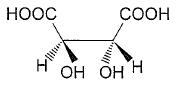[AIEEE 2008]

Solution:

The configuration is done as follow:-
i) We first select the left carbon and hen prioritize its valencies. Since the least prioritised group( Here Hydrogen) is on dashed line, we don’t need any modification.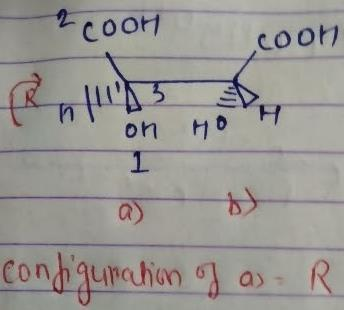So the configuration comes R.
For the other carbon atom, we again prioritise its valencies. Here, the least prioritised group( Here Hydrogen) is not  on the dashed line, we need to put it on the dashed line. So by interchanging H and OH and then applying the configuration, itcomes S.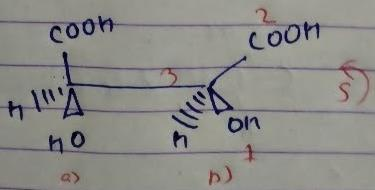But we have done odd times interchange, so the above configuration will be reverse.
The configuration comes out R.
SO the configuration of both carbon atoms are R,R.

QUESTION: 6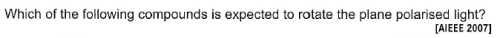Solution:

The correct answer is Option A.

Out of the given four compounds only the first one has a chiral carbon. Hence compound A is optically active and it can rotate the plane polarised light.

QUESTION: 7

Which of the following compounds will have a meso-isomer also?

[AIEEE 2004]

Solution: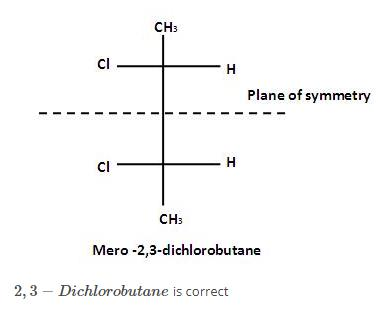QUESTION: 8

amongst the following compounds, the optically active alkane having lowest molar mass is

[AIEEE 2004]

Solution:

Here all compounds except in option c are achiral. So automatically option c is correct.

QUESTION: 9

Consider the following set of molecules.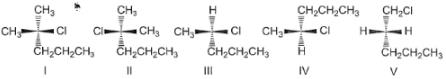The pairs of enantiomers are

Solution:

1 and 2 are non-superimposable mirror images of each other. So, according to the options given, 1 and 2 are enantiomers of each other.

QUESTION: 10

The pair of molecule shown are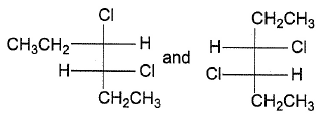Solution:

The configuration of the first compound is (R,S) and that of the second compound is (S,S). And we know that the isomers which are having different configurations at one or more equivalent chiral centers and the same configuration at the remaining chiral centers are called diastereomers.

QUESTION: 11

Pair of the molecule show below are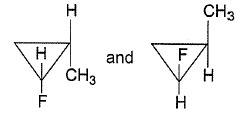Solution:

If we rotate one off the molecule in a plane perpendicular to molecule, then we will get the mirror image of others which are non superimposable. So, they are enantiomers.

QUESTION: 12

Which structures represent(s) diastereomers of I ?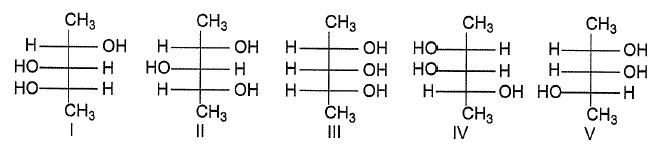Solution:
QUESTION: 13

What is the molecular formula for the alkane of smallest molecular weight which possesses a stereogenic centre?

Solution: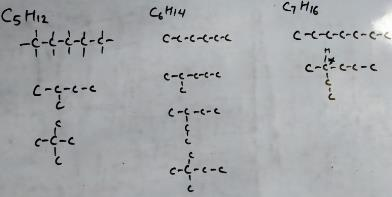I have drawn only skeleton. It can be known that for C5H12 and C6H14, we have no isomerism. For C7H16 we have one isomer. So, the minimum carbon required for an alkane is C7H16.

QUESTION: 14

which compound does not possess a plane of symmetry ?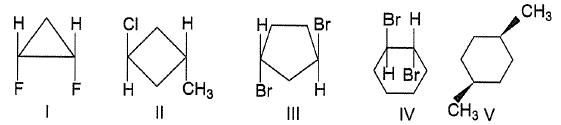Solution:

Compound I has a plane of symmetry along the plane of cyclopropane. Compound II also has a plane of symmetry along the plane which is diagonal to carbon atoms having substituent perpendicular to cyclobutane. However, compounds 3 and 4 have not any plane of symmetry. Compound 5 has a plane of symmetry perpendicular to the plane of cyclohexane dividing outer –CH3. So, the correct option is d

QUESTION: 15

of the compounds, which correspond to the general name dichlorocyclobutane how many are optically active ?

Solution:

The correct answer is option B

2 are optically active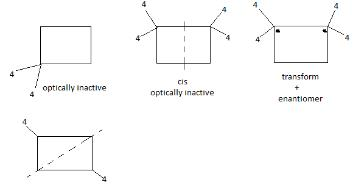QUESTION: 16

Which statement is true about 1,3-dimethyl cyclobutane?

Solution:

The correct Option is B.

Two diastereomeric forms are possible for  1,3-dimethylcyclobutane. These are as shown.

Note:
Diastereomers have different configurations at one or more stereocenters and are not mirror images of each other.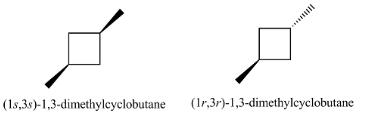QUESTION: 17

Out of following, the alkene that exhibit optical isomerism Is

[AIEEE 2010]

Solution: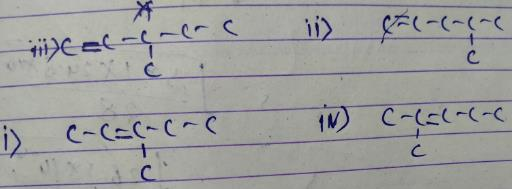We can see that in option iii, we have a chiral centre. So we have an optical somer only in option iii.

QUESTION: 18

How many chiral compounds are possible on monchlorination of 2-methyl butane ?

[AIEEE 2012]

Solution: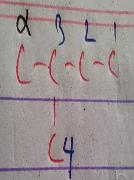The positions are numbered. Note that 4 and α are the same for this purpose.

*Multiple options can be correct
QUESTION: 19

Direction (Q. Nos. 19-22) This section contains 4 multiple choice questions. Each question has four
choices (a), (b), (c) and (d), out of which ONE or  MORE THANT ONE  is correct.

Q.

The correct statement regarding tartaric acid is/are

Solution:

The correct answers are option b,c,d.
The correct statement regarding tartaric acid are:

• b)It has a total of three stereomers
• c)It has only two optically active isomers
• d)It has two pair of diastereomers

*Multiple options can be correct
QUESTION: 20

The correct statement regarding elements of symmetry is/are

Solution:
*Multiple options can be correct
QUESTION: 21

In a pair of diastereomers,

Solution:

Diastereomers are those compounds which have no mirror relationship . So they can be optically active,inactive, dextro or laevo.
However, there is no restriction of no. of carbon atoms. We need at least 1 carbon atom. So option d is incorrect.

*Multiple options can be correct
QUESTION: 22

The correct statement(s) regarding the isomers of compound N-ethyl-N-methyl-l-propanamine is/are

Solution:
QUESTION: 23

Direction (Q. Nos. 23 and 24) This sectionis based on statement I and Statement II. Select the correct answer from the code given below

Q.

Statement I: The following compound is optically inactive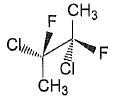Statement II It has an axis of symmertry

Solution:

We know that following are the cases when a compound is Optically Inactive:
1. When it does not have a chiral Carbon Atom eg.: Methyl bromide
2. When it has a Plane or Axis of Symmetry eg.: Tartaric Acid (these compounds are called as Meso Compounds)
3. When it forms a Racemic Mixture eg.: Equimolar mixture of d-Lactic Acid and l-Lactic Acid.
The given compound in the question possesses an Axis of Symmetry (shown in the attached image). Hence, it is Optically Inactive.

QUESTION: 24

Q.

Statement I : The following compound is optically inactive.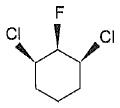Statement II : It has two chiral carbons.

Solution: